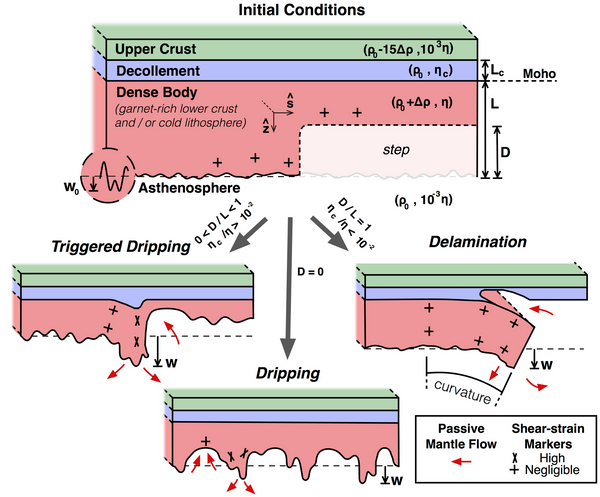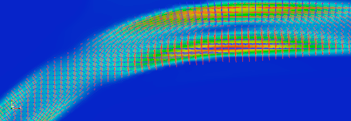# Viscoelasticity in Underworld2

Viscoelastic materials exhibit the properies of both solids and liquids, with deformation rates dependent on both the viscous stress and elastic stress rate.  In a Maxwell viscoelastic material the strain rate $D$ is proportional to the sum of the stress $\tau$ and the stress rate $\dot\tau$,

\begin{equation}
D_{ij} = D^v_{ij} + D^e_{ij} = \frac{\tau_{ij}}{2\eta} + \frac{\dot\tau_{ij}}{2\mu}
\end{equation}

with $\eta$ viscosity and $\mu$ shear modulus. In Underworld this rheology can be implemented using an effective viscosity and an additional force term within the momentum equation,

\begin{equation}
(2\eta_{eff}D_{ij})_{,j} - p_{,i}  = f_i + \frac{\eta_{eff}}{\mu\Delta t_e}\hat\tau_{ij,j}
\end{equation}

with $p$ pressure, $\eta_{eff} = (\eta\Delta t_e)/(\alpha + \Delta t_e)$ the effective viscosity, $\alpha$ the Maxwell relaxation time, $\Delta t_e$ the elastic time step and $\hat\tau$ the stress history term. See Farrington et al (2014) for full details.

### Viscoelasticity in simple shear

A uniform visc

oelastic material undergoes simple shear at a constant rate until $t = 4$. The shearing velocity is then taken to zero with the stress decaying with time.

The graph above shows the shear component of the stress history term from equation (2) for a range of Maxwell relaxation times (alpha).  See the viscoelastic example for details, my_uw/doc/2_11_ViscoelasticityInSimpleShear.ipynb

### An objective stress rate tensor

The stress history term (stress rate tensor) is carried on particles that can be advected and rotated through time by the velocity field.  To maintain objectivity it must be both advected and rotated for the current reference frame.  This can be acheived using the Jaumann stress rate, defined by $\hat\tau_{ij} = \dot\tau_{ij} + \tau_{ik}w_{kj}+\tau_{jk}w_{ki}$ with $w$ the vorticity tensor. If significant rotation occurs the use of the Jaumann stress rate is encouranged. There are other terms which may be added to this definition accounting for other changes in reference frame including stretching. However, including these additional terms couples stress and pressure, adding a considerable complication that is not required for mathematical consistency.

For full details regarding the background, numerics and use in geodynamic modelling please see Farrington et al (2014) and the references within.

Farrington, R. J., L.-N. Moresi, and F. A. Capitanio (2014), The role of viscoelasticity in subducting plates, Geochem. Geophys. Geosyst., 15, 4291–4304

## Discussion

Related Posts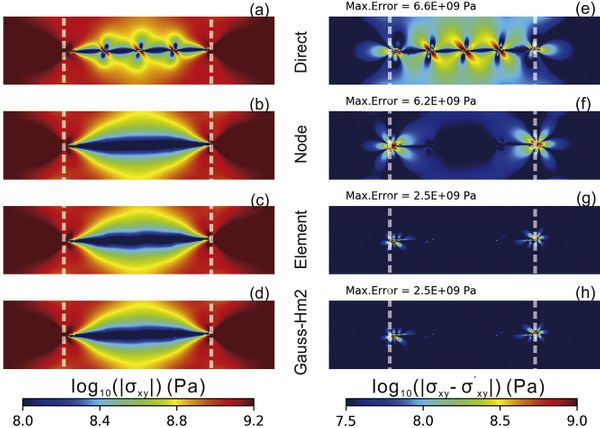## Stress recovery in Underworld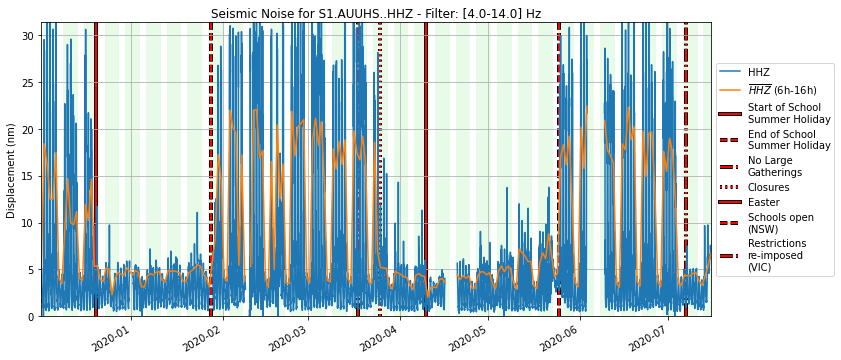## Australian cities are quiet during lockdown. Earthquake scientists are making the most of it## Underworld publication news: Crustal thickness anomalies in stable continents.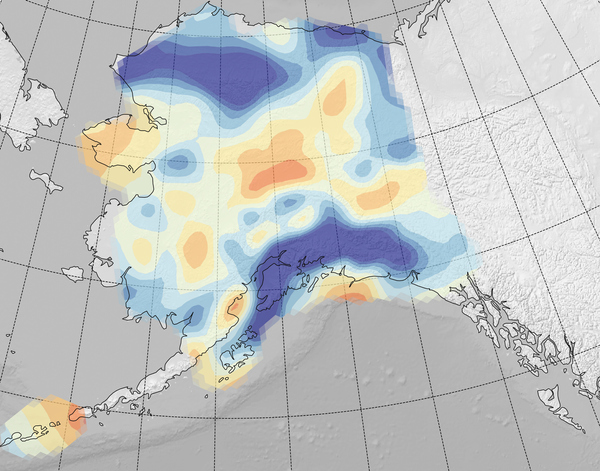## Alaska Moho Model (Reproducible research with containers)## Craton Formation and the Onset of Plate Tectonics